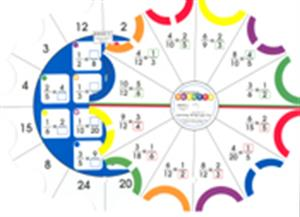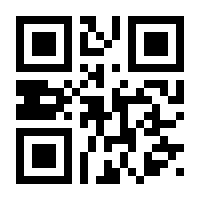See more:Most PopularMonthly Special

This set includes 144 challenges (12 cards with 12 challenges per card), giving students practice dealing with identification of fractional parts of wholes and of groups, identification of fractions along a number line, estimation using fractions, identifying equivalent fractions, simplifying fractions, comparing fractional differences, ordering, adding and subtracting fractions with like denominators, and adding and subtracting mixed fractions with like denominators.

This product meets the National Council of Teachers of Mathematics (NCTM) standards. Each card is SELF-CORRECTING.

These cards develop Numeration skills.

Card 1 -- Identify fractional part of a whole.
Card 2 -- Identify fractional part of a group.
Card 3 -- Identify fractions on a number line.
Card 4 -- Estimate with fractions.
Card 5 -- Identify equivalent fractions.
Card 6 -- Simplify fractions.
Card 7 -- Compare fractions.
Card 8 -- Order fractions.
Card 9 -- Add fractions with like denominators.
Card 10 -- Subtract fractions with like denominators.
Card 11 -- Add mixed fractions with like denominators.
Card 12 -- Subtract mixed numbers with like denominators..

To be used in conjunction with the "Learning Palette Base", part number LP-B100.This book contains scannable QR codes for additional features. See above description for details.
Price:
\$
9.99
\$
9.99
\$
Author:
Illustrator:
Series:
Learning Palette - Math
ISBN:
LP-M412
Binding:
Pages:
Size:
8 1/4 in diameter